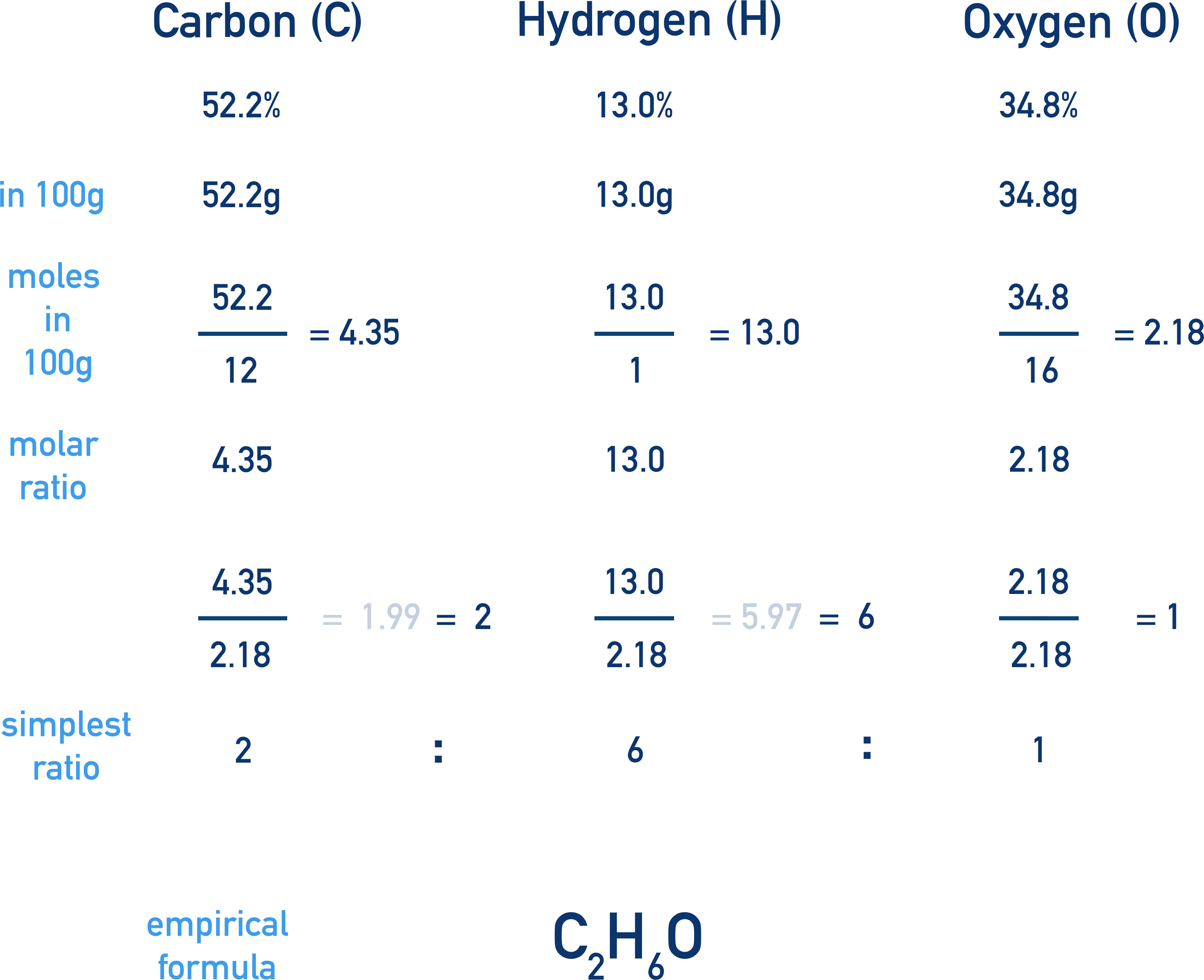Video Tutorial Empirical Formula

Quick Notes Empirical Formula

• An empirical formula is the simplest, whole number ratio of different atoms within a compound.
• Empirical formulas can be determined by using the percentage by mass of each atom type within the compound.
• For ionic compounds, the empirical formula is the same as the ionic formula (for example, NaCl).

Full Notes Empirical Formula (how to calculate)

An empirical formula is the simplest, whole number ratio of different atoms within a compound.

Empirical formulas can be determined by using the percentage by mass of each atom type within the compound. This isn’t as confusing as it sounds!

Percentage by mass refers the percentage of the compound that is made up of each atom type. See Percentage by Mass.

If we are given the percentage by mass data for a particular compound, the empirical formula can be found by converting the mass ratio of elements to a molar ratio.

## Calculating Empirical Formula

For example, let’s find the empirical formula for a compound that is made up of 82.7% carbon and 17.3% (percentage by mass data).The first thing to do is to convert the mass ratio to a molar ratio. This is because we can only compare numbers of atoms within a compound if we use moles (mass is different for every element!).

The easiest way to do this is two convert the percentages to grams - almost like pretending we have a 100g sample.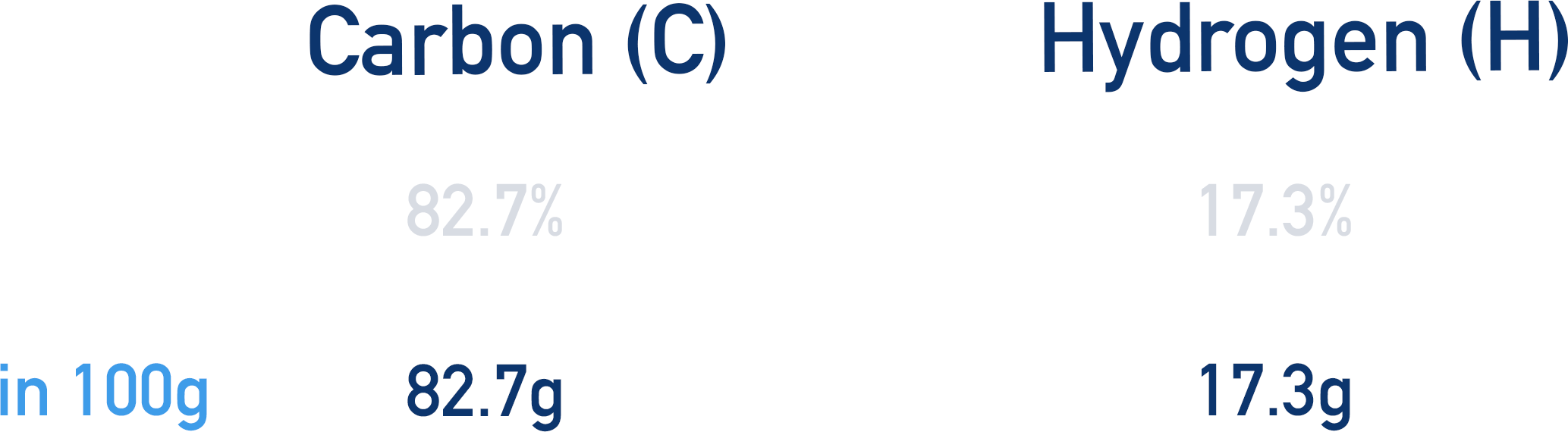If 82.7% of the compound is carbon, then in 100g of the compound, there would be 82.7g of carbon.

If 17.3% of the compound is hydrogen, then in 100g of the compound, there would be 17.3g of hydrogen.

We can now use the masses to find the moles of each element that would be in 100g.

(number of moles = mass / Mr)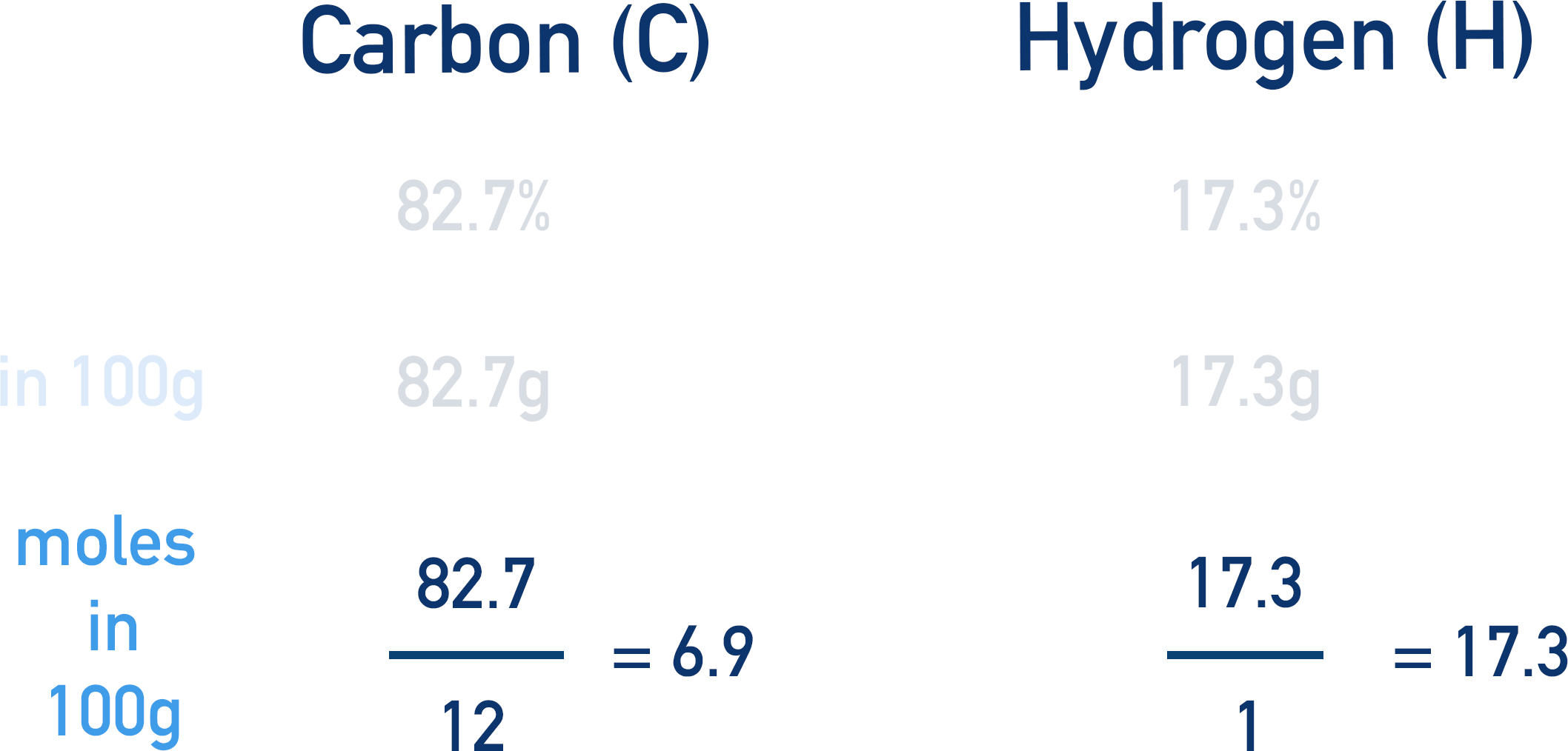We now have a molar ratio!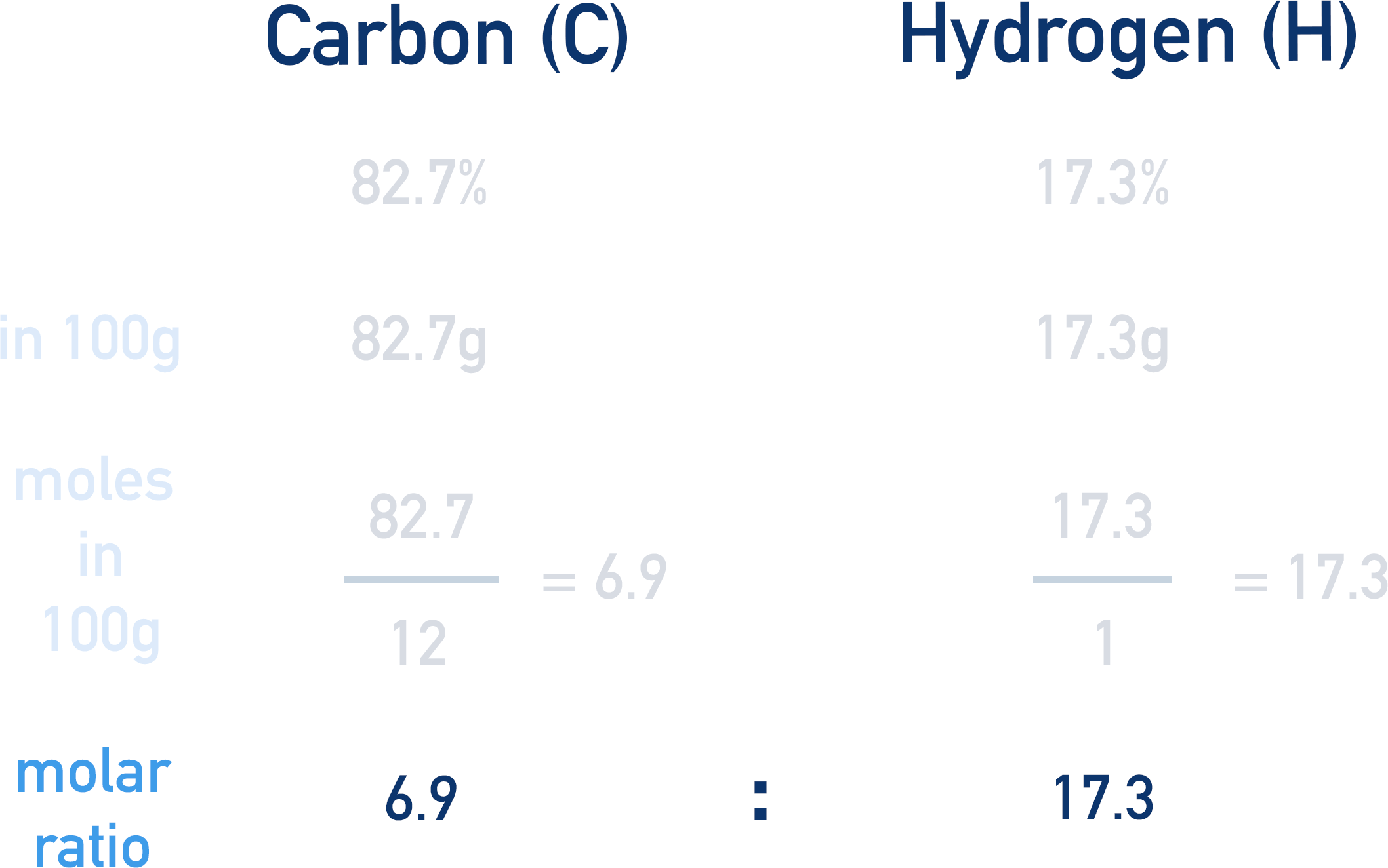To make this a simpler ratio, we divide the smallest number by itself and the larger number(s) by the smallest number.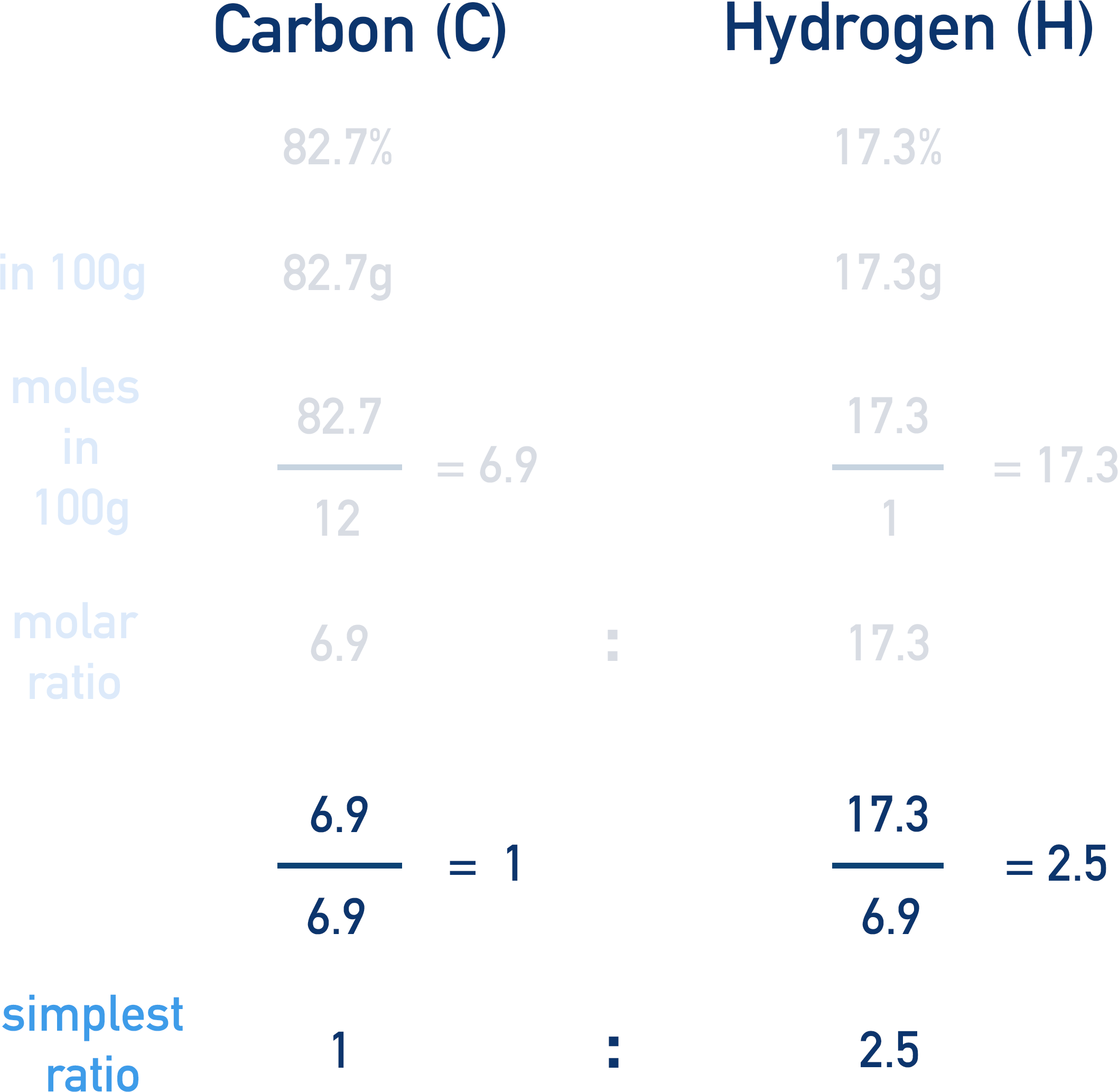In this case, carbon has the lowest number (6.9 moles)

6.9 moles / 6.9 = 1 mole of carbon

17.3 moles / 6.9 = 2.5 moles of hydrogen

The ratio is now simpler, 1 : 2.5 , but it still isn’t a whole number ratio.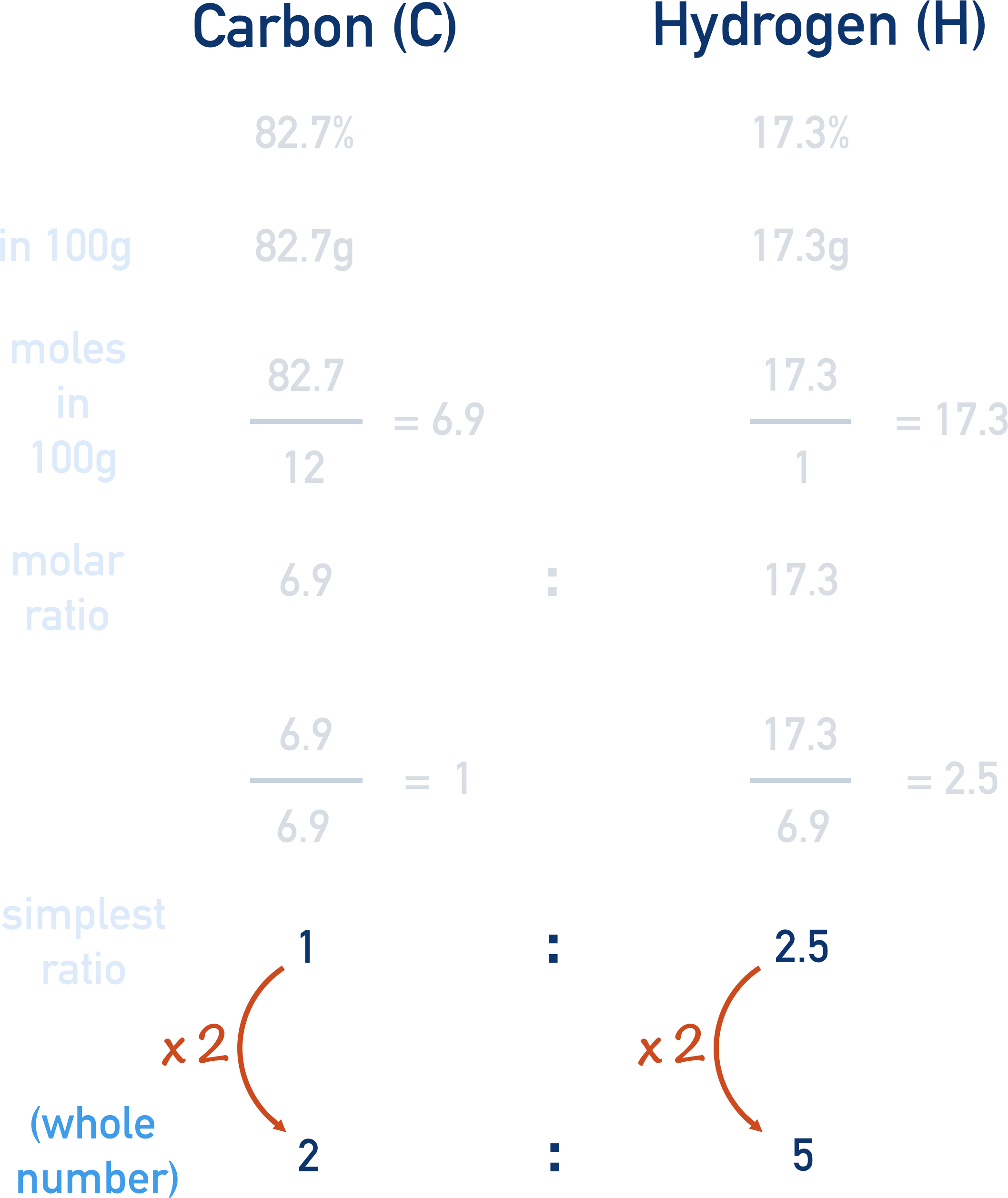1 : 2.5 can be multiplied by 2 to give the ratio 2 : 5, this is now the simplest, whole number ratio.

The empirical formula of the compound would be C2H5.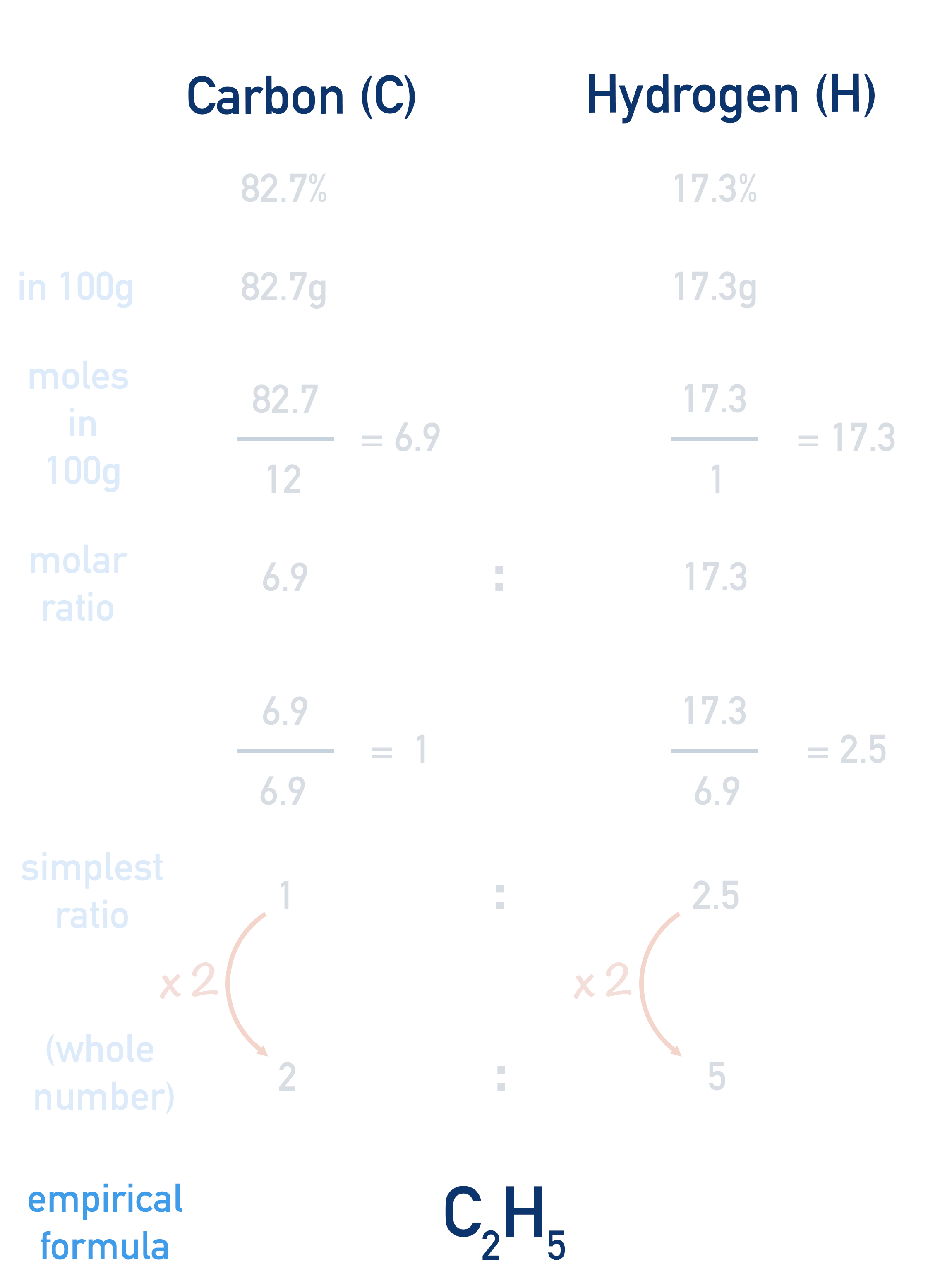Another example is shown below, without the explanation above, so you can see how the workings are laid out.

Find the empirical for the compound with a composition by mass of C 52.2%, H 13.0% and O 34.8%